# Online Digital Electronics Test - Digital Electronics Test 8Loading Test...

Instruction:

• This is a FREE online test. DO NOT pay money to anyone to attend this test.
• Total number of questions : 20.
• Time alloted : 30 minutes.
• Each question carry 1 mark, no negative marks.
• DO NOT refresh the page.
• All the best :-).

1.

Convert the following decimal number to 8-bit binary.
35

A.
 000100102B.
 000100112C.
 001000112D.
 0010001022.

A byte has 8 bits.

A.
 TrueB.
 False3.

If the output of an enable circuit must be HIGH when the circuit is disabled, should an AND gate or an OR gate be used for the circuit?

A.
 AND gateB.
 OR gateC.
 Either gate can be used.D.
 Neither gate can be used.4.

A variable is a symbol used to represent a logical quantity that can have a value of 1 or 0.

A.
 TrueB.
 False5.

The terminal marked A on the CTR block in the given figure is the SET terminal.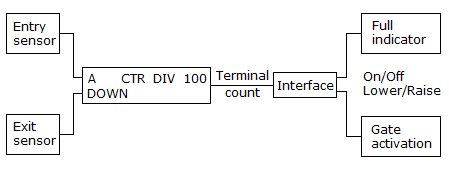A.
 TrueB.
 False6.

RAMs must have a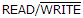input, in addition to data in.

A.
 TrueB.
 False7.

The most widespread application of ROMs is in the storage of data and program codes that must be available on power-down in the microprocessor-based systems. These data and program codes are called firmware because they are firmly stored in hardware.

A.
 TrueB.
 False8.

The Boolean sum of the four product terms is called the sum-of-products.

A.
 TrueB.
 False9.

The ________ is the most popular standard logic device family today.

A.
 TTLB.
 CMOSC.
 ECLD.
 None of the above10.

What is the result of taking more samples during the quantization process?

A.
 More errors in the analog-to-digital conversionB.
 More bit requirementsC.
 More accurate signal representationD.
 More bit requirements and more accurate signal representation11.

Polling is the process of pausing the normal sequence of instructions to cause the processor to execute a separate set of I/O instructions instead.

A.
 TrueB.
 False12.

If [A] = 1011 1010, [B] = 0011 0110, and [C] = [A] • [B], what is [C 4..2] in decimal?

A.
 1B.
 2C.
 3D.
 413.

Refer to the display multiplexer given below. The MSD display is blank, while the LSD seems to be OK. The input and output lines to the 74157 and 7449 are checked with a scope and can be seen changing levels. The A input on the 74139 also changes; however, the 0 output on the 74139 is always LOW and the 1 output is always HIGH. The B and EN inputs on the 74139 are always LOW. What could cause the problem and what should be done to correct it?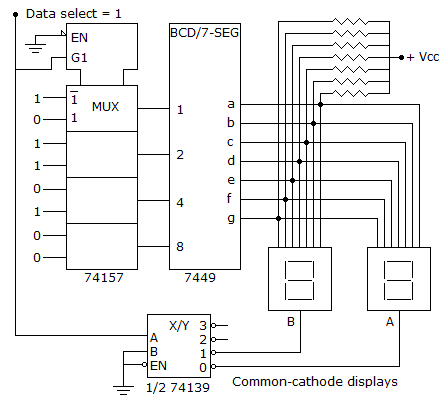A.
 The 74139 is defective; replace it.B.
 The EN pin on the 74139 could have a bad connection; check the connection.C.
 The 74139 is defective and must be replaced; or the EN pin on the 74139 could have a bad connection, which should be checked.D.
 The MSD display is bad; replace it.14.

In order to select data input I5 of a 74151 eight-line multiplexer, the select inputs must be ________.

A.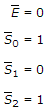B.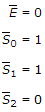C.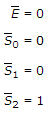D.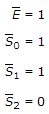15.

In the keypad HDL encoder, as long as all columns are high the ring counter is enabled and counting.

A.
 TrueB.
 False16.

Which of the following characterizes an analog quantity?

A.
 Discrete levels represent changes in a quantity.B.
 Its values follow a logarithmic curve.C.
 It can be described with a finite number of steps.D.
 It has a continuous set of values over a given range.17.

Sample-and-hold circuits in A/D converters are designed to:

A.
 sample and hold the output of the binary counter during the conversion processB.
 stabilize the comparator's threshold voltage during the conversion processC.
 stabilize the input analog signal during the conversion processD.
 sample and hold the D/A converter staircase waveform during the conversion process18.

A binary-weighter resistor DAC is practical only up to a resolution of ________.

A.
 10 bitsB.
 2 bitsC.
 8 bitsD.
 4 bits19.

PMOS and NMOS ________.

A.
 represent MOSFET devices utilizing either P-channel or N-channel devices exclusively within a given gateB.
 are enhancement-type CMOS devices used to produce a series of high-speed logic known as 74HCC.
 represent positive and negative MOS-type devices, which can be operated from differential power supplies and are compatible with operational amplifiersD.
 None of the above20.

A single Schmitt trigger inverter is all that is needed to build a simple astable multivibrator.

A.
 TrueB.
 False